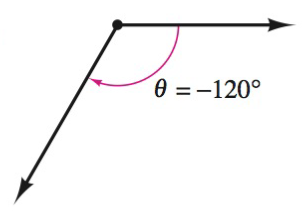Chapter 8.1, Problem 3E### Calculus: An Applied Approach (Min...

10th Edition
Ron Larson
ISBN: 9781305860919

#### Solutions

Chapter
Section### Calculus: An Applied Approach (Min...

10th Edition
Ron Larson
ISBN: 9781305860919
Textbook Problem
1 views

# Finding Coterminal Angles In Exercises 1-6, determine two coterminal angles in degree measure (one positive and one negative) for the angle. See Example 1.To determine

To calculate: The two coterminal angles in degree measure, in which one is positive and another is negative with the help of provided diagram,Explanation

Given Information:

The provided angle is 120°, which is shown below as,

Formula used:

The angle measuring θ has same initial and terminal sides as the angle measuring 360θ, such angles are coterminal angle.

Calculation:

Draw diagram for coterminal angles and provided angle for θ=120°.

From above diagram, let θ+ be the positive coterminal angle and θ be the negative coterminal angle

### Still sussing out bartleby?

Check out a sample textbook solution.

See a sample solution

#### The Solution to Your Study Problems

Bartleby provides explanations to thousands of textbook problems written by our experts, many with advanced degrees!

Get Started

#### Evaluate the expression sin Exercises 116. 323

Finite Mathematics and Applied Calculus (MindTap Course List)

#### True or False: A straight (simple) substitution may be used to evaluate 2+lnxxdx.

Study Guide for Stewart's Single Variable Calculus: Early Transcendentals, 8th

#### 0 1 π It does not exist.

Study Guide for Stewart's Multivariable Calculus, 8th